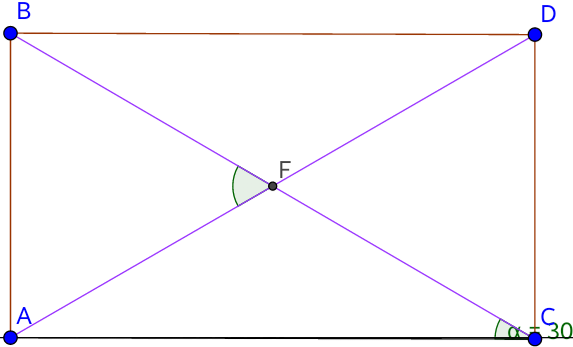# Angel angleIn the above figure, $ABDC$ is a rectangle where $\angle{BCA} = {30}^\circ$. Find the measure of $\angle{BFA}$ in degrees.

×